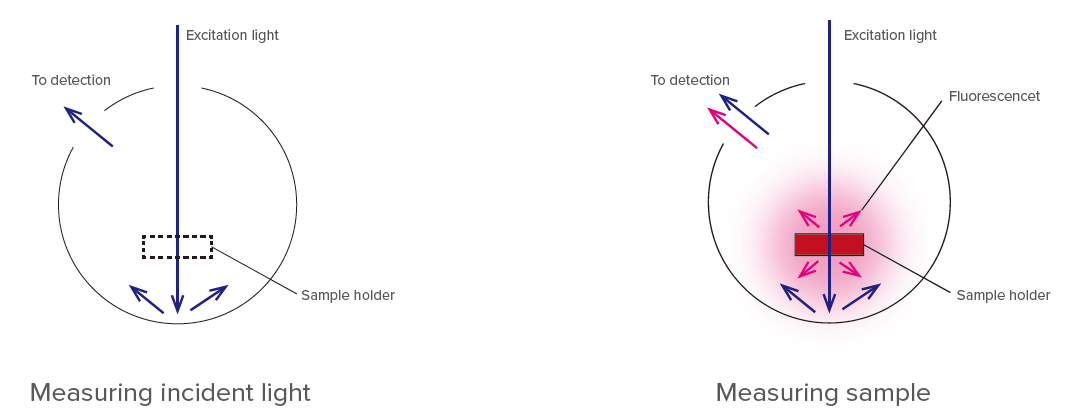# Fluorescence quantum yield measurement

March 10, 2021

## Introduction

Fluorescence quantum yield is defined as the ratio of the number of photons emitted from sample as fluorescence to the number of photons in the excitation light absorbed. Absolute method and relative method are known as measuring methods.

## Relative method

Relative method can calculate quantum yield of unknown sample by comparing the intensity of standard fluorescence with unknown sample, and accordingly, the relative method is easier to get the results of quantum yield.

[Calculation method]
In order to calculate relative quantum yield, the following parameters are required.

(1)Quantum yield of standard sample: Φst
(2)Absorbances at excitation wavelength for the standard sample (st) and the unknown sample (x): Ast, Ax
(3)Areas of fluorescence spectrum with spectral correction for the standard sample (st) and the unknown sample (x): Fst, Fx
(4)Average refractive index values in the wavelength range to calculate the area of fluorescence spectrum for the standard sample (st) and the unknown sample (x): nst, nx
*) Its information is required when the solvent of the standard sample is different from that of unknown sample
(5)Dilution ratio for the standard sample (st) and the unknown sample (x) respectively: Dst, Dx
*) Its information is required when the standard or unknown samples for fluorescence spectrum measurement are diluted

By using the parameters above, relative quantum yield of unknown sample, Φx is shown by the following equation.## Absolute method

The absolute method allows to detect all the fluorescence from the sample and integrates using integrating sphere. Therefore, its method enables more accurate quantum yield measurement.

[Measurement method]

(1)Measuring incident light
Set the cell with solvent on the sample cell holder in integrating sphere, and measure spectrum of incident light (In case of the solid sample, confirm nothing is set on the sample cell holder in the integrating sphere, and measure spectrum of the incident light). Obtained peak area is defined as area from incident light, S0 (equivalent number of photons in the incident light).
(2)Measuring sample
Set the sample on the sample holder, and measure scattering and fluorescence spectra of the sample. Obtained excitation wavelength peak area is defined as area scattered from sample, S1 (equivalent number of photons which were not absorbed), and peak area in the emission wavelength range is defined as area emitted from sample, S2.(3) Calculating quantum yield
Calculate in accordance with the following.
• Sample absorption (%) = (S0S1)/S0 × 100
• External quantum yield (%) = S2/S0 × 100
• Internal quantum yield (%) = S2/(S0S1 ) × 100JASCO can provide the fluorescence quantum yield measurement system (relative method or absolute method), and also propose the proper solution to meet the user’s requirement.FP-8550 Spectrofluorometer